# JFreeChart

This category contains 9 nodes.

#####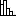Bar Chart (JFreeChart)

Displays a bar chart for columns with nominal values.

#####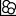Bubble Chart (JFreeChart)

This node creates a bubble-chart visualization. It uses two variables for the position of a data point and a third one is mapped to the radius of the […]

#####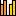GroupBy Bar Chart (JFreeChart)

Displays a bar chart for all ready grouped columns with nominal values. You can use the GroupBy-Node to gain the required columns.

#####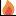HeatMap (JFreeChart)

A HeatMap maps each value onto a color scale and visualizes it in a rectangle. It can be used to show lists of numbers and distance matrices.

#####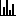Histogram Chart (JFreeChart)

Display a histogram chart.

#####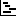Interval Chart (JFreeChart)

Displays a interval chart.

#####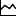Line Chart (JFreeChart)

Display a line plot using x,y data points and connects them using a line. The colors are chosen from the second input table.

#####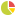Pie Chart (JFreeChart)

Node to display a pie chart.

#####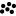Scatter Plot (JFreeChart)

The node visualize the input data by putting each data point to the position on the plot (X and Y value).